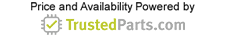Industrial & Medical Technology

# How to control a DC Motor with an Arduino

12 March 2020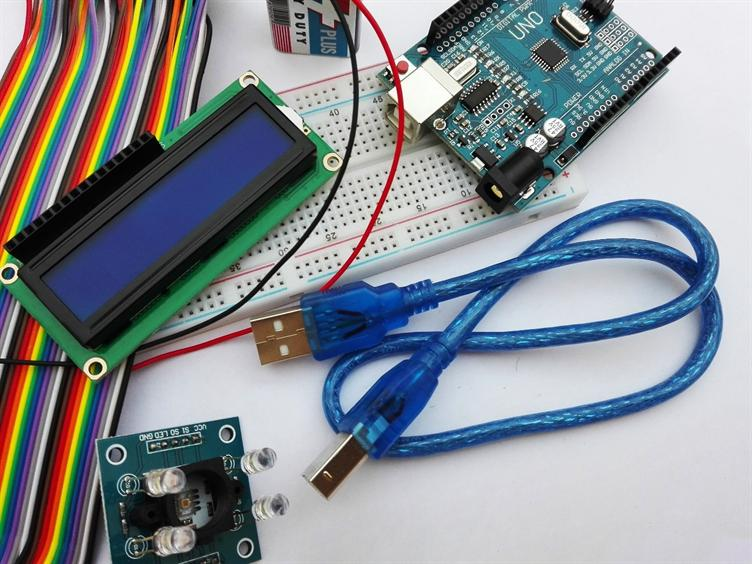Introduction

By controlling the motor, we mean controlling its speed and direction of rotation. We can control the motor using the Arduino and the L298N driver. Before we learn how to control a DC motor with the Arduino, we need to understand some basic concepts of motor control. The speed of the DC motor can be easily controlled by adjusting the input voltage supplied to the motor. We can control the input voltage with a PWM signal.

PWM DC motor control

Using pulse-width modulation (PWM), we can apply the average voltage value to our device, adjusting its value by turning the signal on and off at high speed. The duty cycle is defined as the amount of time during which the signal is turned on, compared with the amount of time during which the signal is turned off. An increase in the duty cycle means that the average voltage is high and therefore the motor speed will be high. The concept of the duty cycle can be understood more clearly using figure 1.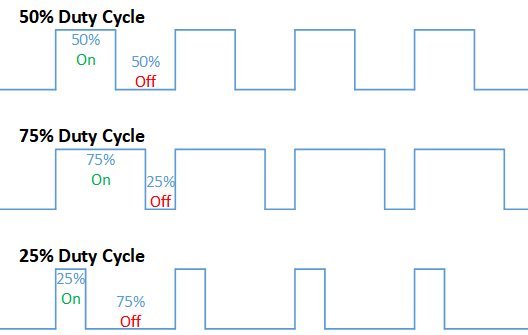Figure 1: The concept of Duty Cycle

Arduino has a PWM output pin, and we can just connect this PWM output to a transistor base (or to the gate of a MOSFET). One end of the power supply will be connected to the motor terminal, and the other motor terminal will be connected to the collector. The emitter of the transistor will be connected to ground. We can control the motor speed by controlling the PWM signal of the Arduino. The MOSFET acts like a switch that turns on and off with a PWM signal from Arduino. Arduino cannot control the motor directly, because the signal has low power, in addition, Arduino will burn out due to excessive current consumption of the motor. Thus, we will use this low power signal only to turn the transistor / field effect transistor on and off. In this way, we can control the speed of the DC motor using the Arduino. The circuit diagram is shown in Figure 2.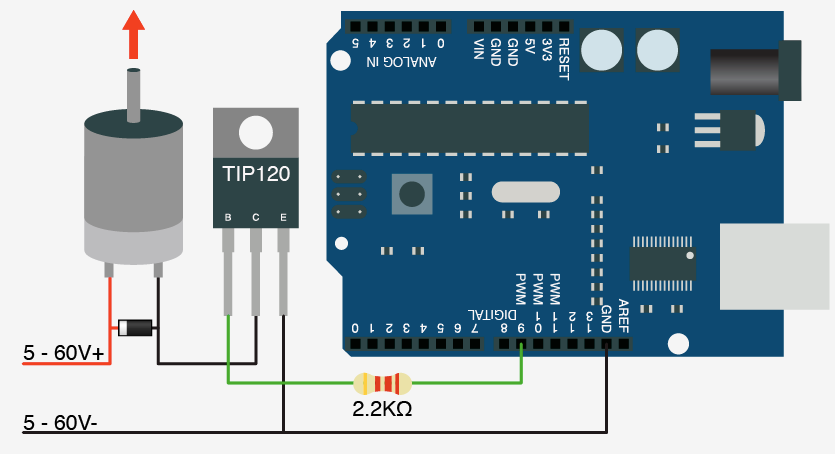Figure 2: Speed controlling of motor using Arduino

H-Bridge DC motor control

Next, we want to control the direction of rotation of the motor. We can achieve this by changing the direction of the current through the motor. For this purpose, we will use the H-Bridge scheme. This circuit can be formed by using 4 transistors or MOSFETs, and the motor will be in the center. The overall scheme will look H-shaped, therefore, known as the H-Bridge scheme. We will use signals from Arduino to activate two transistors simultaneously to control the direction of rotation of the motor. The H-bridge scheme is shown in figure 3.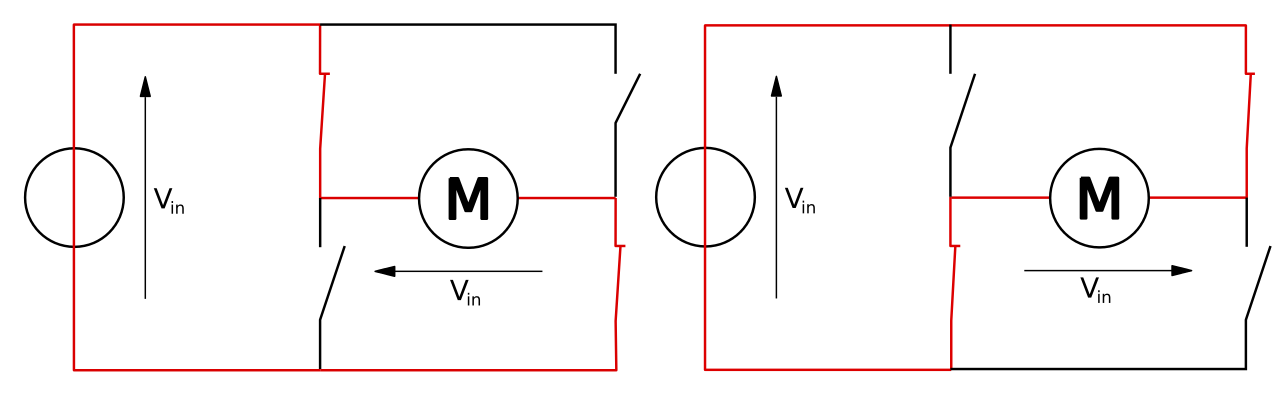Figure 3: The H-Bridge Scheme

Now we understand two methods that can be used to control the speed and direction of the DC motor. The next step is to develop a circuit that will allow us to fully control the DC motor. We will use the Arduino for the PWM signal, and also to turn the transistors of H-Bridge on and off.

We can design our own H-bridge according to the voltage and current consumed by the motor. We can choose transistors / MOSFETs according to motor size. All current passing through the motor will also pass through the transistors, so if no wise choice is made, the transistors / MOSFETs will burn out. There are several built-in standard drivers also available on the market, and we will use the L298N in our circuit.

L298N driver

This driver allows us to control the speed and direction of two DC motors. It has two H-bridge circuits, one for each motor. It can be used for DC motors from 5 to 35 V with a peak current of not more than 2 A. The L298N driver is shown in figure 4.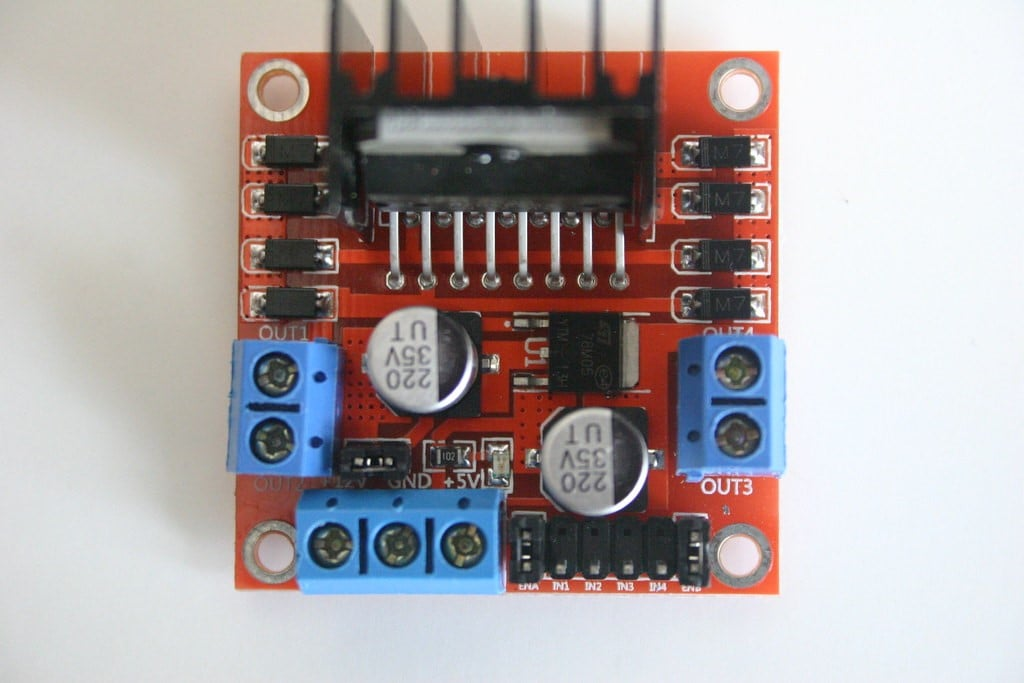You need to understand how this module works, and also look at its pinout. We can connect two motors to the motor terminals A and B. The VCC power for the motors can be connected to the screw terminal block; the ground terminal and the 5V terminal are also connected to this block. We can use the jumper on the board if the VCC is greater than 12V. This will protect the circuit from damage due to high voltage.

Enable A and B are control inputs used to control the speed of the DC motor. The motor will turn on and run at maximum speed with a jumper. We can remove the jumper and use the PWM signal to control the motor speed. We can turn off the motor by connecting these contacts to ground. Figure 5 highlights each pin with a label.

The direction of rotation of motor A is controlled from inputs 1 and 2, and motor B is controlled from inputs 3 and 4. We use these contacts to control the H-bridges of IC L298N. The motor will move forward when input 1 is low and input 2 is high, and the motor will move back when input 1 is high and input 2 is low. When both inputs are the same, this means that the brake and motor will stop.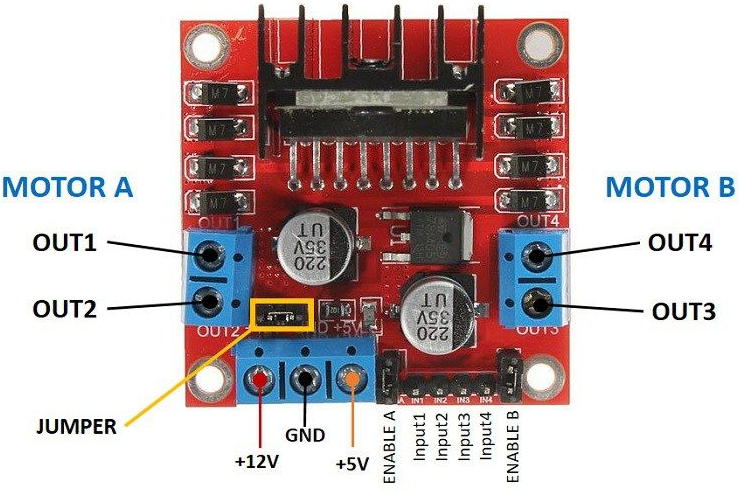Figure 5: L298N Driver Pinout

Arduino and L298N

We are ready to consider a practical example in which we will use the Arduino and L298N IC to control a DC motor. We will use the PWM signal from Arduino to control the motor speed, and we will change the PWM signal using a potentiometer.

Required components

Following are the components which we will need to make this circuit.

1. Arduino

2. L298N Driver

3. 12V High Torque DC Motor

5. Push Button

Arduino code

Here’s the Arduino code:

#define enA 9

#define in1 6

#define in2 7

#define button 4

int rotDirection = 0;

int pressed = false;

void setup() {

pinMode(enA, OUTPUT);

pinMode(in1, OUTPUT);

pinMode(in2, OUTPUT);

pinMode(button, INPUT);

// Set initial rotation direction

digitalWrite(in1, LOW);

digitalWrite(in2, HIGH);

}

void loop() {

int pwmOutput = map(potValue, 0, 1023, 0 , 255); // Map the potentiometer value from 0 to 255

analogWrite(enA, pwmOutput); // Send PWM signal to L298N Enable pin

pressed = !pressed;

}

delay(20);

// If button is pressed - change rotation direction

if (pressed == true & rotDirection == 0) {

digitalWrite(in1, HIGH);

digitalWrite(in2, LOW);

rotDirection = 1;

delay(20);

}

// If button is pressed - change rotation direction

if (pressed == false & rotDirection == 1) {

digitalWrite(in1, LOW);

digitalWrite(in2, HIGH);

rotDirection = 0;

delay(20);

}

}

Description

We will need some variables in the program, so the first step is to define them. We set the initial direction of rotation of the motor in setup mode. We also set contact modes in this section. This section will be executed only once.

The loop section will execute recursively. We start by reading the value from the potentiometer, which will be from 0 to 1023, then we will convert this value to 0 to 255, since the PWM works in this range. We will send a single PWM to the Enable pin of the L298N chip using the analogWrite () function, which will drive the motor.

Each time the push button is pressed, it changes the direction of rotation of the motor. So, we will check whether the button is pressed and, if so, we will change the states of the inputs so that the direction changes.

Motor driver troubleshooting tips

To ensure that your drive and motor are working properly, we will introduce some of the most common problems that cause a malfunction.

The most common question:

“Drive does not run the motor”

As an engineer, it is very important to look at the right place to find the cause of the failure. Here are some important things to look at when troubleshooting a drive.

Line power: Sometimes the drive does not receive the main power. Therefore, before looking at a drive to find a malfunction, you must make sure that the drive is actually receiving power.

Proper motor connection: The motor is not always connected to the driver output. Sometimes a resistance is connected, so before checking the driver, make sure that the output devices are working. For example, if the motor resistance shows a very high value, which is an open, then the drive is OK, and you just need to check the devices outside the driver.

Make sure the drive is sending the signal to the motor: If there is voltage at the output of the driver, this means that the driver is actually sending a signal to the motor, and a fault is present in the motor.

Control signal: Ensure that the controller actually sends a control command signal to the drive to start the motor. For example, if we use a potentiometer to control the speed, measure the command signal between counter-clockwise and the central terminal to find out if there is a linear increase or decrease in the command signal when the potentiometer changes position.

Applications

There are many applications of controlled DC motors. These motors are used where adjustable speed and low-speed torque are required, such as conveyors and turntables. A DC motor is used in many industrial machines for dynamic braking and reversing. Their linear torque curve, quick stop, reverse and acceleration make them a very good choice when designing something innovative.